# ¿Are critical k-forests tight?

 Importance: Medium ✭✭
 Author(s): Strausz, Ricardo
 Subject: Graph Theory » Hypergraphs
 Keywords: heterochromatic number
 Prize: A cognac at Coyoacan
 Posted by: Dino on: September 1st, 2007
Conjecture

Letbe a-uniform hypergraph. Ifis a critical-forest, then it is a-tree.

We say that a hypergraph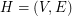is a-graph if it is-uniform, and denote its order byand its size by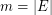.

Laszlo Lovasz introduced the following concept: a-graphis said to be a-forest if for every edgethere exists a-colouing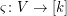such that; that is, such that only the edgereceives thecolours in its vertices. Clearly a-forest is simply a forest in the usual sense (i.e., an acyclic graph). Lovasz proved that

Theorem   A-forest has size at most.

On the other hand, Victor Neumann-Lara introduced the following invariant: the heterochromatic number of a-graphis the minimum number of colourssuch that, in every colouring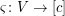there is an edge wich receives different colours in each of its vertices; that is, there existssuch that. If the heterochromatic number and the rank are equal, the hypergraph is said to be tight. Clearly a-graph is tight if and only if it is connected. A tight-forest is called a-tree.

I can prove the following

Theorem   If a-forest has size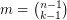then it is tight — and therefore a-tree.

Finally, we say that a-forest is critical if no edge can be added to it without loosing the property of being a-forest; it is maximal (in size) with such a property. Observe that there are critical-forests of size, whenever.

So, the conjecture is to motivate the question: ¿are critical-forests tight?

## Bibliography

* indicates original appearance(s) of problem.

### This has been solved.

See http://arxiv.org/abs/1109.3390